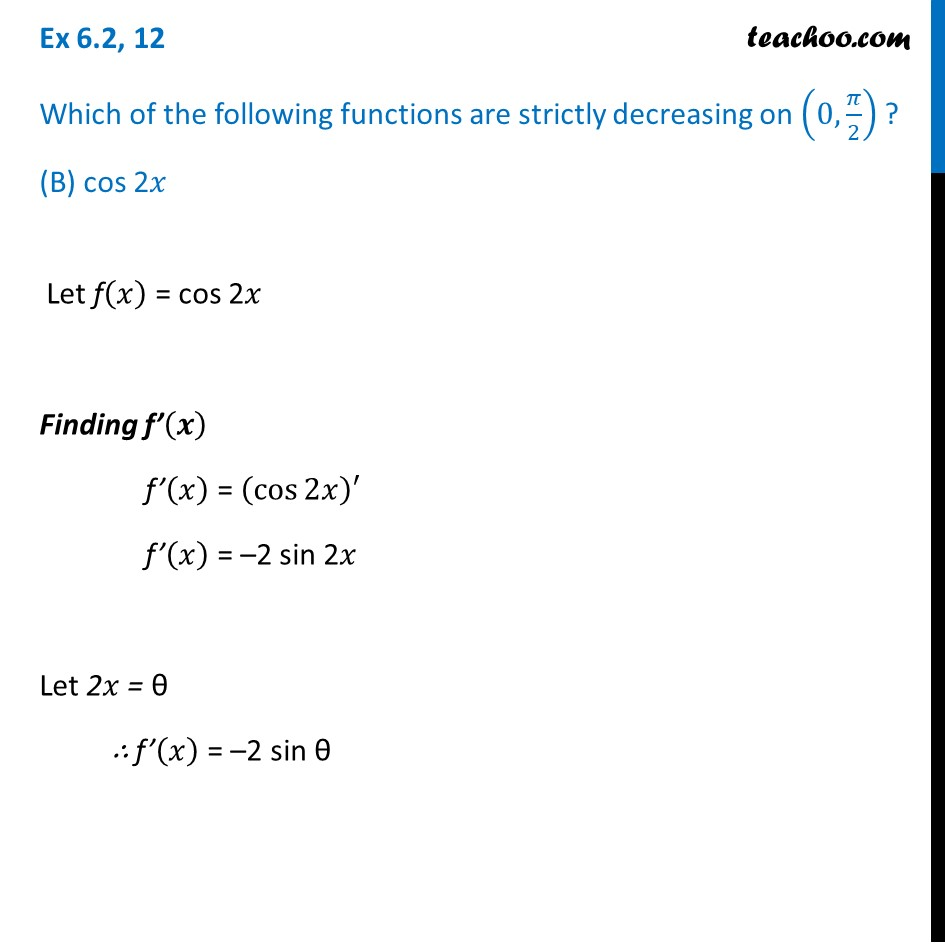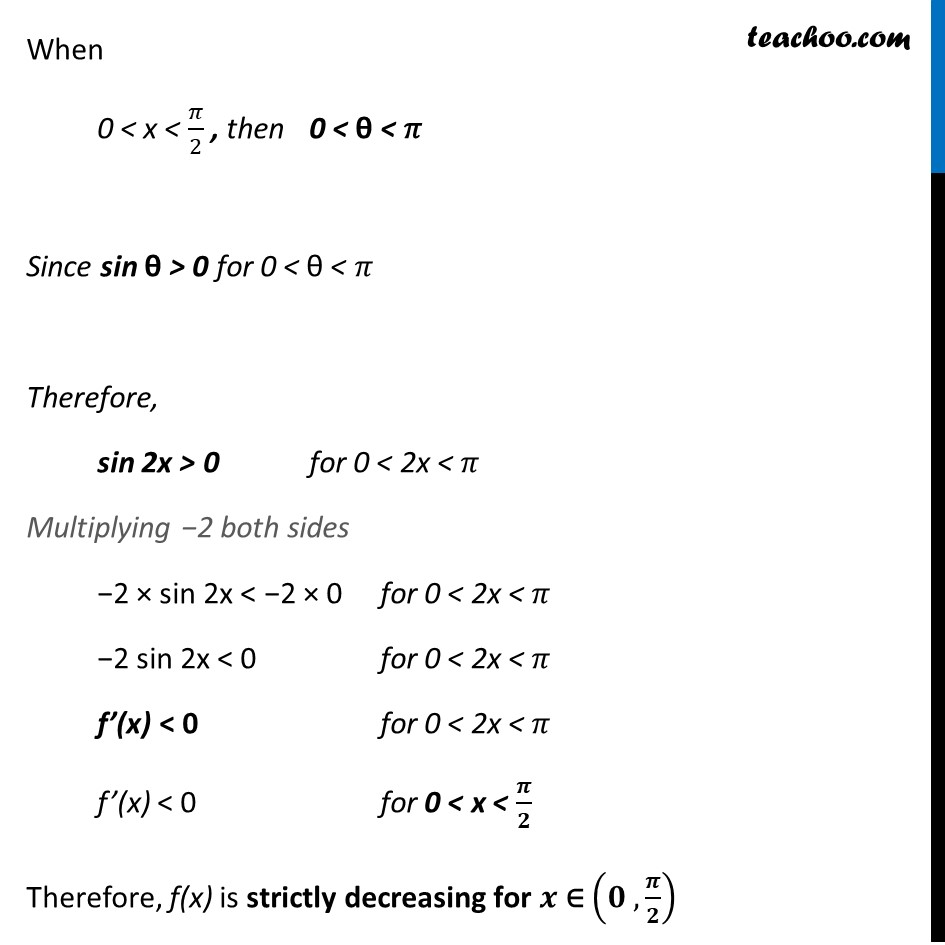Ex 6.2

Chapter 6 Class 12 Application of Derivatives
Serial order wiseLearn in your speed, with individual attention - Teachoo Maths 1-on-1 Class

### Transcript

Ex 6.2, 12 Which of the following functions are strictly decreasing on (0,𝜋/2) ? (B) cos 2𝑥 Let f(𝑥) = cos 2𝑥 Finding f’(𝒙) f’(𝑥) = (cos⁡2𝑥 )′ f’(𝑥) = –2 sin 2𝑥 Let 2𝑥 = θ ∴ f’(𝑥) = –2 sin θ When 0 < x < 𝜋/2 , then 0 < θ < 𝜋 Since sin θ > 0 for 0 < θ < 𝜋 Therefore, sin 2x > 0 for 0 < 2x < 𝜋 Multiplying −2 both sides −2 × sin 2x < −2 × 0 for 0 < 2x < 𝜋 −2 sin 2x < 0 for 0 < 2x < 𝜋 f’(x) < 0 for 0 < 2x < 𝜋 f’(x) < 0 for 0 < x < 𝝅/𝟐 Therefore, f(x) is strictly decreasing for 𝒙 ∈ (𝟎 , 𝝅/𝟐)